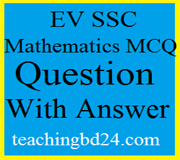# EV SSC MCQ Question Ans. Simple Simultaneous Equations with Two Variables

EV SSC MCQ Question Ans. Simple Simultaneous Equations with Two Variables. If four quantities are such that the ratio of first and second quantities is equal to the ratio of third and fourth quantities, those four quantities form a proportion. If a, b, c, d are four such quantities, we write a : b = c : d . The four quantities of proportion need not be of same kinds. The two quantities of the ratio are to be of the same

## EV SSC MCQ Question Ans. Simple Simultaneous Equations with Two Variables###If four quantities are such that the ratio of first and second quantities is equal to the ratio of third and fourth quantities, those four quantities form a proportion. If a, b, c, d are four such quantities, we write a : b = c : d . The four quantities of proportion need not be of same kinds. The two quantities of the ratio are to be of the same the kind only.

teachingbd24.com is such a website where you would get all kinds of necessary information regarding educational notes, suggestions and questions’ patterns of school, college and madrasahs. Particularly you will get here special notes of physics that will be immensely useful to both students and teachers. The builder of the website is Mr. Md. Shah Jamal Who has been serving for 30 years as an Asst. Professor of BAF Shaheen College. He expects that this website will meet up all the needs of Bengali version learners /students. He has requested concerned both students and teachers to spread this website home and abroad.